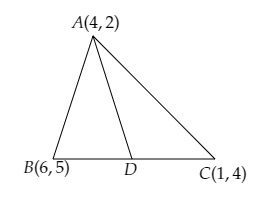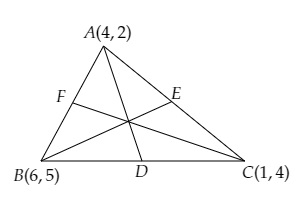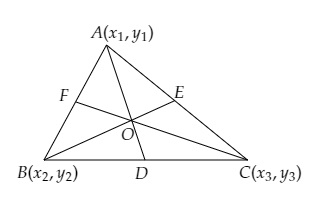# Let $A(4, 2), B(6,5)$ and $C(1, 4)$ be the vertices of $Ã¢Ë†â€ ABC$.(i) The median from $A$ meets $BC$ at $D$. Find the coordinates of the point $D$.(ii) Find the coordinates of the point $P$ on $AD$, such that $AP : PD = 2 : 1$.(iii) Find the coordinates of points $Q$ and $R$ on medians $BE$ and $CF$ respectively, such that $BQ : QE = 2 : 1$ and $CR : RF = 2 : 1$.(iv) What do you observe?[Note: The point which is common to all the three medians is called centroid and this point divides each median in the ratio 2: 1](v) If $A(x_1, y_1), B(x_2, y_2)$ and $C(x_3, y_3)$ are the vertices of $Ã¢Ë†â€ ABC$, find the coordinates of the centroid of the triangles.

To do:

We have to find:

(i) The coordinates of point D.

(ii) Find the coordinates of the point $P$ on $AD$, such that $AP : PD = 2 : 1$.

(iii) Find the coordinates of points $Q$ and $R$ on medians $BE$ and $CF$ respectively, such that $BQ : QE = 2 : 1$ and $CR : RF = 2 : 1$.

(iv) What do we observe

[Note: The point which is common to all the three medians is called centroid and this point divides each median in the ratio of 2: 1]

(v) If $A(x_1, y_1), B(x_2, y_2)$ and $C(x_3, y_3)$ are the vertices of $∆ABC$, find the coordinates of the centroid of the triangles.

Solution:

(i) $D$ is the mid-point of BC.This implies,

Using the mid-point formula, we get,

Coordinates of $D=(\frac{6+1}{2}, \frac{5+4}{2})$

$=(\frac{7}{2},\frac{9}{2})$

The coordinates of the point $D$ are $(\frac{7}{2},\frac{9}{2})$ .

(ii) $D$ is the mid-point of BC.This implies,

Using the mid-point formula, we get,

Coordinates of $D=(\frac{6+1}{2}, \frac{5+4}{2})$

$=(\frac{7}{2},\frac{9}{2})$

$AP : PD = 2 : 1$.

Using the section formula, we get,

$(x,y)=(\frac{m x_{2}+n x_{1}}{m+n}, \frac{m y_{2}+n y_{1}}{m+n})$

The coordinates of $\mathrm{P}$ are $(\frac{2 \times \frac{7}{2}+1 \times 4}{1+2}, \frac{2 \times \frac{9}{2}+1 \times 2}{1+2})$

$=(\frac{7+4}{3}, \frac{9+2}{3})$

$=(\frac{11}{3}, \frac{11}{3})$

The coordinates of $\mathrm{P}$ are $(\frac{11}{3}, \frac{11}{3})$.

(iii) $D$ is the mid-point of BC.This implies,

Using the mid-point formula, we get,

Coordinates of $D=(\frac{6+1}{2}, \frac{5+4}{2})$

$=(\frac{7}{2},\frac{9}{2})$

Similarly,

Coordinates of $E=(\frac{4+1}{2}, \frac{2+4}{2})$

$=(\frac{5}{2},3)$

Coordinates of $F=(\frac{4+6}{2}, \frac{2+5}{2})$

$=(5,\frac{7}{2})$

$AP : PD = 2 : 1$.

Using the section formula, we get,

$(x,y)=(\frac{m x_{2}+n x_{1}}{m+n}, \frac{m y_{2}+n y_{1}}{m+n})$

The coordinates of $\mathrm{P}$ are $(\frac{2 \times \frac{7}{2}+1 \times 4}{1+2}, \frac{2 \times \frac{9}{2}+1 \times 2}{1+2})$

$=(\frac{7+4}{3}, \frac{9+2}{3})$

$=(\frac{11}{3}, \frac{11}{3})$

$BQ : QE = 2 : 1$

The coordinates of  $\mathrm{Q}$ are $(\frac{2 \times \frac{5}{2}+1 \times 6}{1+2}, \frac{2 \times 3+1 \times 5}{1+2})$

$=(\frac{5+6}{3}, \frac{6+5}{3})$

$=(\frac{11}{3}, \frac{11}{3})$

$CR : RF = 2 : 1$

The coordinates of  $\mathrm{R}$ are $(\frac{2 \times 5+1 \times 1}{1+2}, \frac{2 \times \frac{7}{2}+1 \times 4}{1+2})$

$=(\frac{10+1}{3}, \frac{7+4}{3})$

$=(\frac{11}{3}, \frac{11}{3})$

We observe that the coordinates of $P, Q$ and $R$ are the same. $P, Q$ and $R$ coincide with each other.

Medians of the sides of a triangle pass through the same point which is called the centroid of the triangle.

Therefore, $(\frac{11}{3},\frac{11}{3})$ is the centroid of the triangle ABC.

The coordinates of $\mathrm{Q}$ and $\mathrm{R}$ are $(\frac{11}{3}, \frac{11}{3})$.

(iv) Since, the coordinates of $\mathrm{P}, \mathrm{Q}$ and $\mathrm{R}$ are $(\frac{11}{3}, \frac{11}{3}, \frac{11}{3})$ this shows that medians of $P, Q$ and $R$ intersect each other at centroid of the $\triangle {ABC}$.

(v)We know that,

Coordinates of the centroid of a triangle are $(\frac{Sum\ of\ abscissa}{3}, \frac{Sum\ of\ ordinates}{3})$

Therefore,

The coordinates of the centroid of triangle ABC are,

$(\frac{x_1+x_2+x_3}{3}, \frac{y_1+y_2+y_3}{3})$

The coordinates of the centroid of triangle ABC are $(\frac{x_1+x_2+x_3}{3}, \frac{y_1+y_2+y_3}{3})$.

Similarly,

The coordinates of the centroid of triangle ABC whose vertices are $A(4, 2), B(6,5)$ and $C(1, 4)$ are

$(\frac{4+6+1}{3}, \frac{2+5+4}{3})=(\frac{11}{3}, \frac{11}{3})$

Updated on: 10-Oct-2022

19 Views